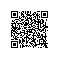# 连续 3 年最受欢迎：Rust，香！### 一 性能对比

#### 静态编译（或者打包）后生成的二进制大小对比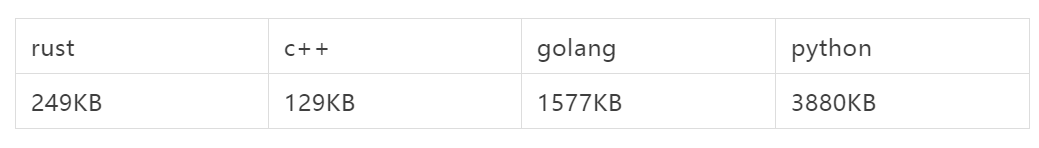#### 运行速度对比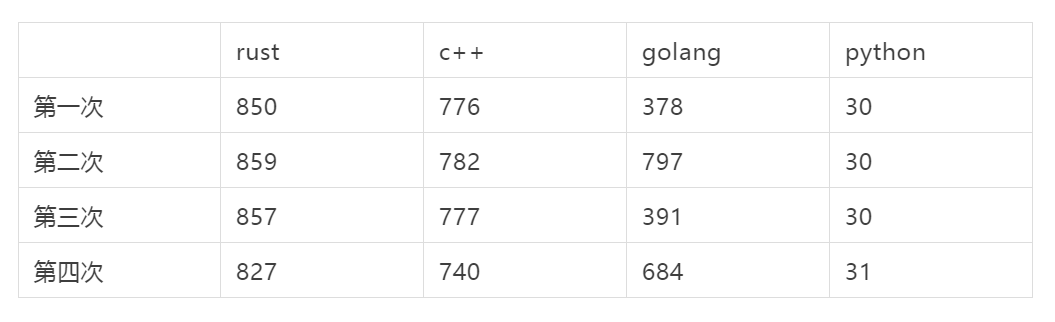#### 内存消耗对比（粗略计算）#### CPU消耗对比（粗略计算）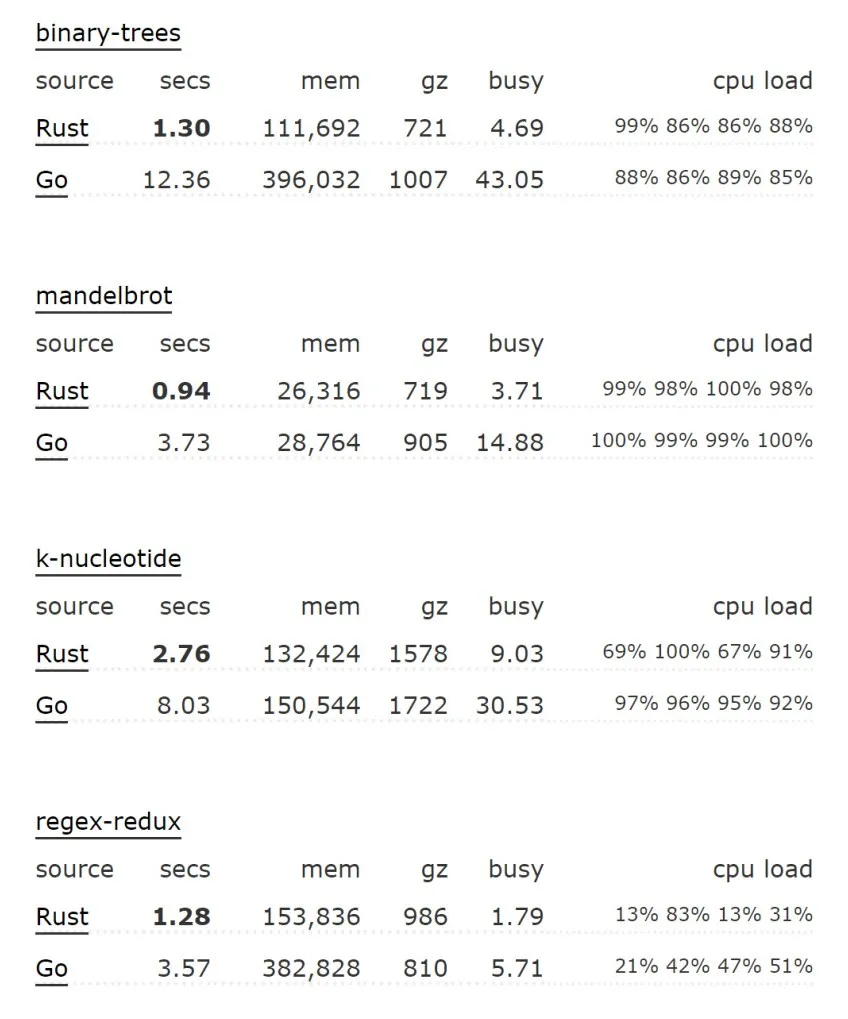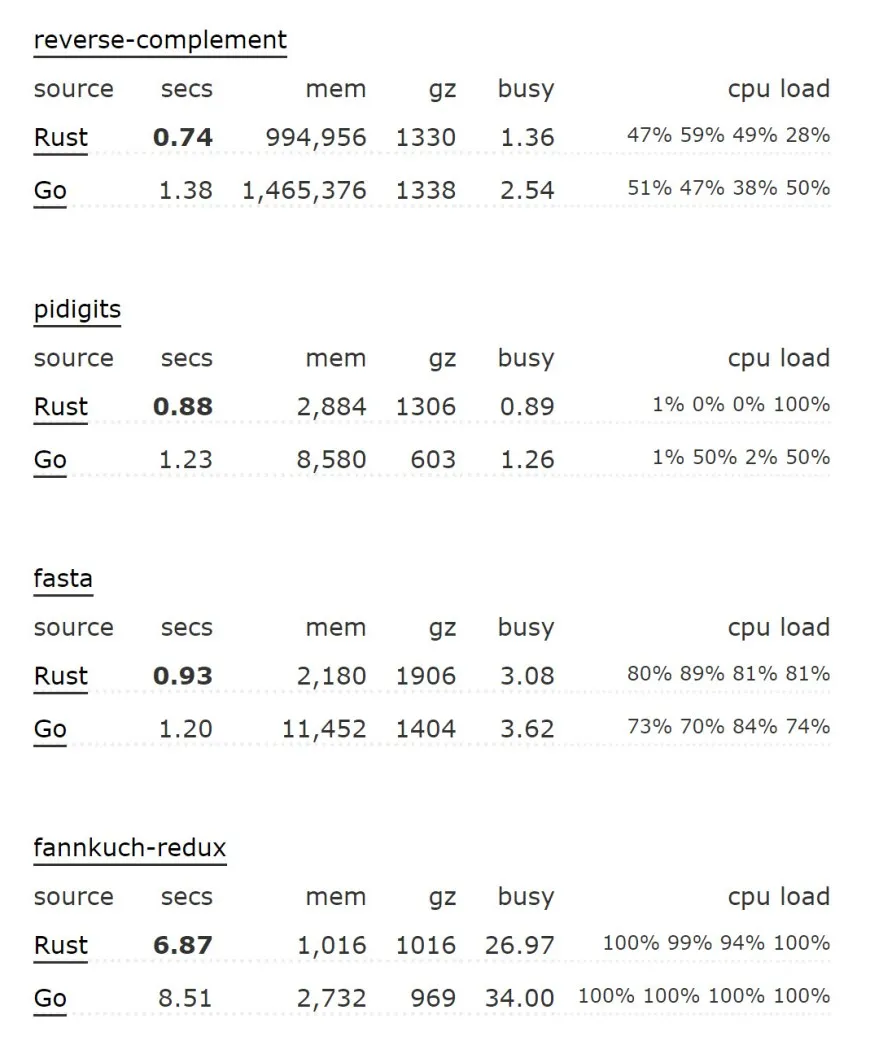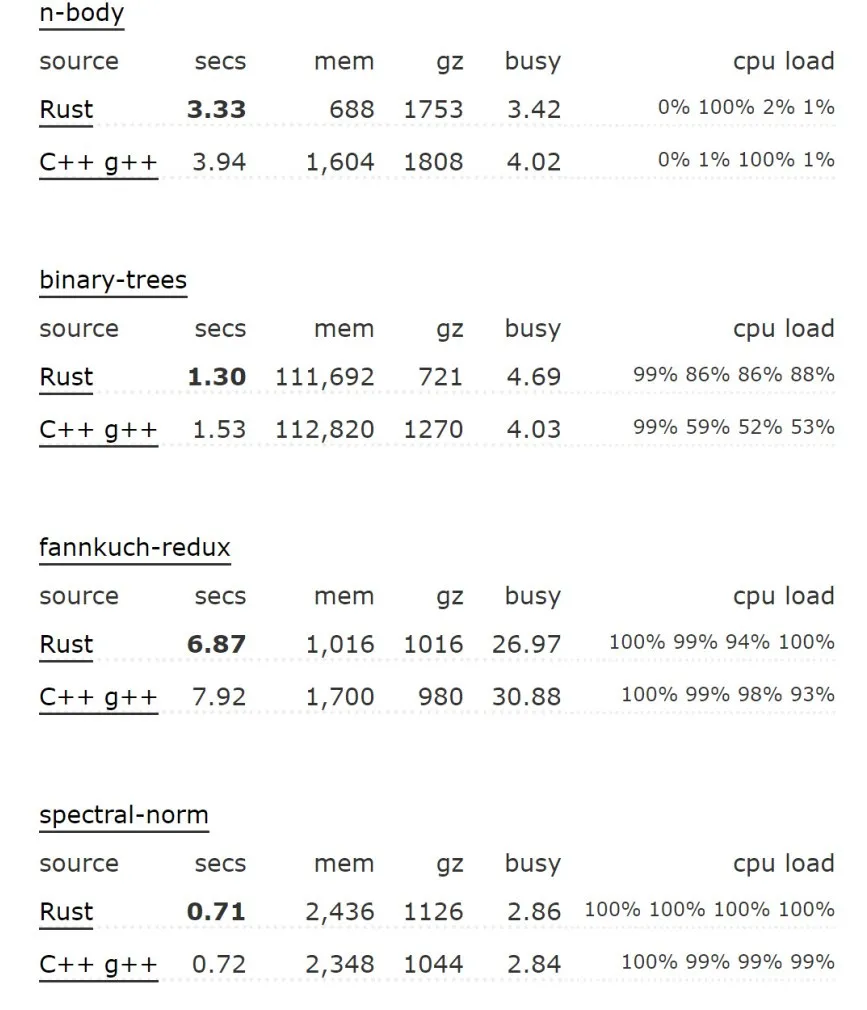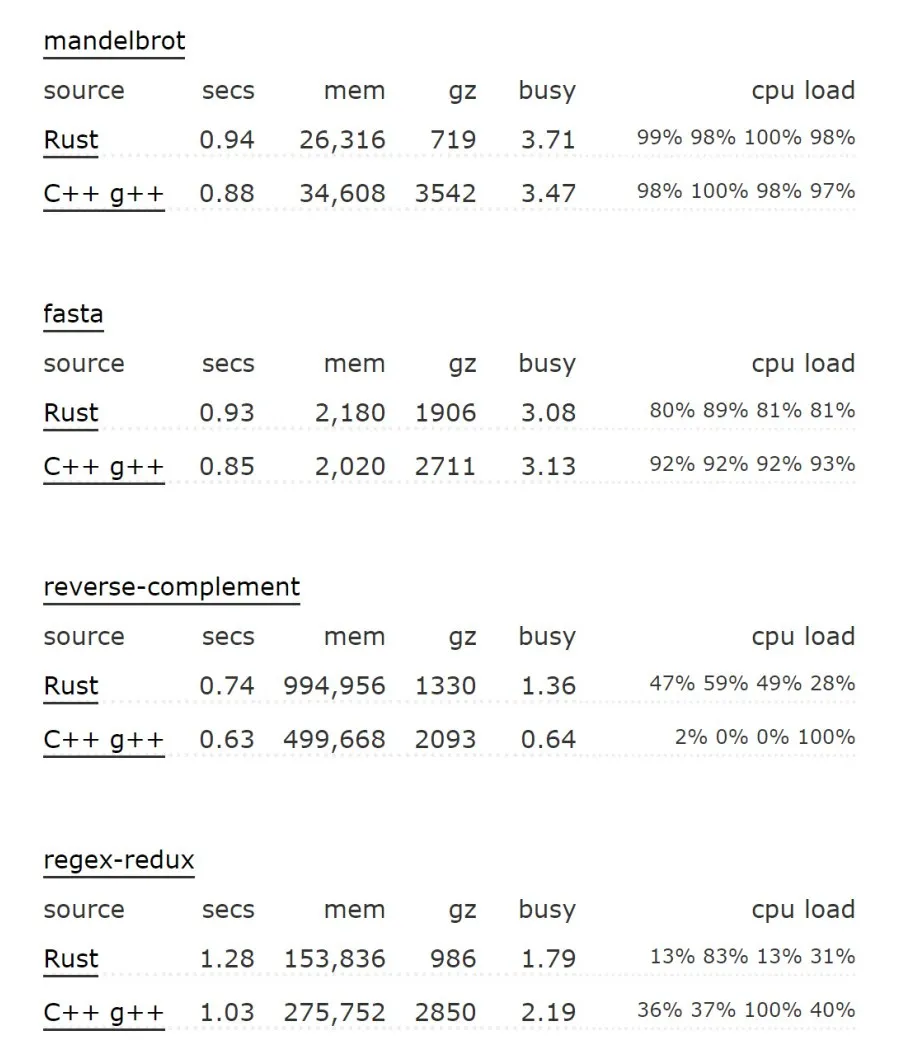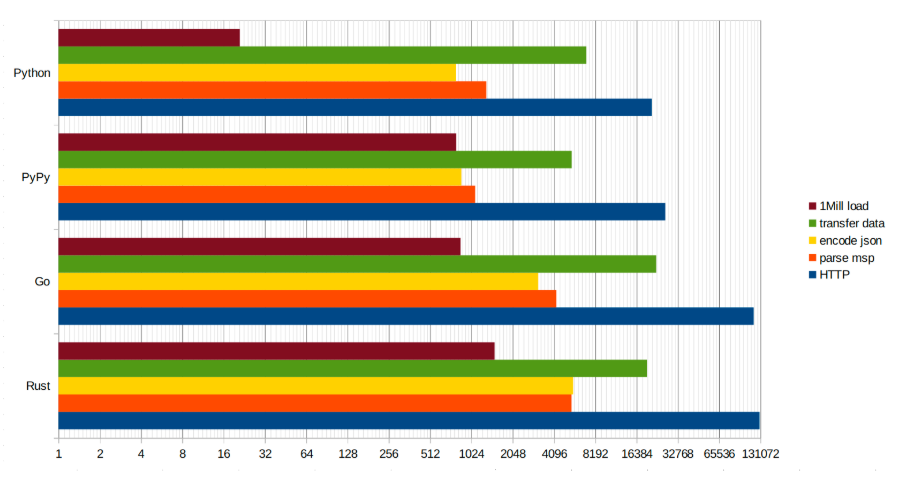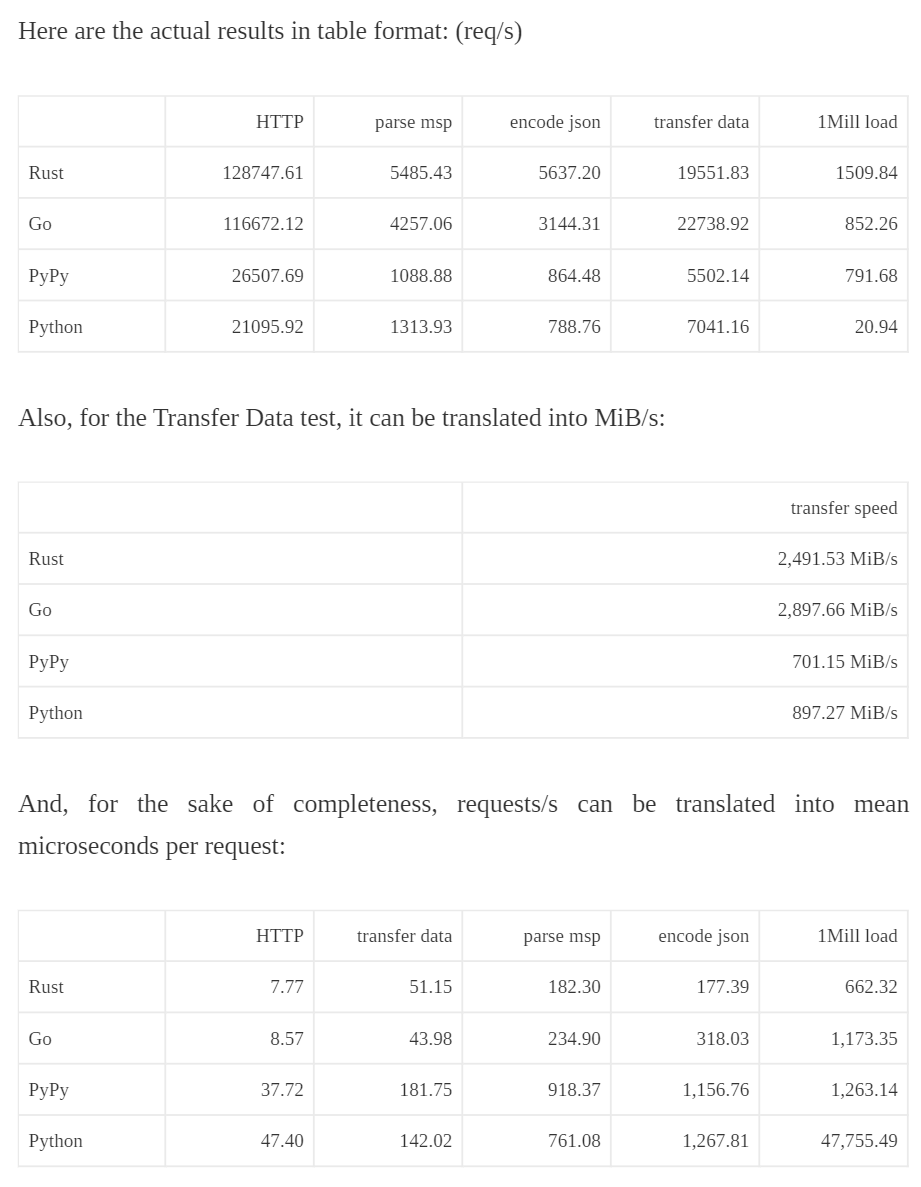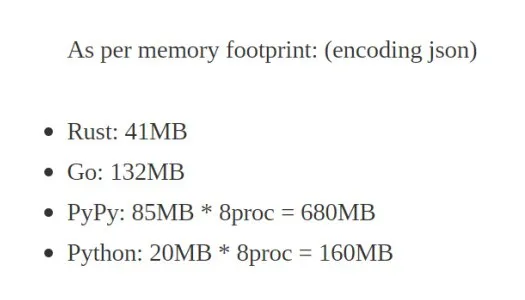### 二 内存安全性

Rust 最重要的特点就是可以提供内存安全保证，而且没有额外的性能损失。在传统的系统级编程语言（ C/C＋＋） 的开发过程中，经常出现因各种内存错误引起的崩溃或bug ，比如空指针、野指针、内存泄漏、内存越界、段错误、数据竞争、迭代器失效等，血泪斑斑，数不胜数；内存问题是影响程序稳定性和安全性的重大隐患，并且是影响开发效率的重大因素；根据google和微软 两大巨头的说法，旗下重要产品程序安全问题70%由内存问题引发， 并且两个巨头都用利用Rust语言来解决内存安全问题的想法。Rust语言从设计之初就把解决内存安全作为一个重要目标，通过一系列手段保证内存安全，让不安全的潜在风险在编译阶段就暴露出来。接下来根据自己粗浅的理解，简单介绍Rust解决内存安全的手段有哪些。

#### 1 所有权规则

1）Rust 中每一个值或者对象都有一个称之为其 所有者（owner）的变量。

let obj = String::from("hello");

obj是String对象的所有权变量。

2）值或对象有且只能有一个所有者。

3）当所有者离开作用域，所有者所代表的对象或者值会被立即销毁。

4）赋值语句、函数调用、函数返回等会导致所有权转移，原有变量会失效。

fn main() {
let s = String::from("hello");
let s1 = s; //所有权发生了转移，由s转移给s1
print!("{}",s); //s无效，不能访问，此句编译会报错
}
fn test(s1:String){
print!("{}",s1);
}

fn main() {
let s = String::from("hello");
test(s); //传参，所有权发生了转移
print!("{}",s); //此处s无效，编译报错
}

Rust的所有权规则保证了同一时刻永远只有一个变量持有一个对象的所有权，避免数据竞争。

#### 2 借用规则

fn test(s1:&String){
print!("{}",s1);
}

fn main() {
let s = String::from("hello");
test(&s); //传参，注意只是传递了引用，所有权还归属于s
print!("{}",s); //此处s依然有效，可以访问
}
fn main() {
let s = String::from("hello");
let s1 = &s; //s1借用s，所有权还归属于s
print!("{}",s); //此处s依然有效，可以访问
print!("{}",s1); //此处s1和s指向同一个对象
}

fn main() {
let s = String::from("hello");
change(&s);

}

fn change(some_string: &String) {
some_string.push_str(", world");
}

error[E0596]: cannot borrow immutable borrowed content *some_string as mutable
--> error.rs:8:5
|
7 | fn change(some_string: &String) {
|                        ------- use &mut String here to make mutable
8 |     some_string.push_str(", world");
|     ^^^^^^^^^^^ cannot borrow as mutable

fn main() {
let mut s = String::from("hello");
change(&mut s);

}

fn change(some_string: &mut String) {
some_string.push_str(", world");
}

let mut s = String::from("hello");
let r1 = &mut s;
let r2 = &mut s;

error[E0499]: cannot borrow s as mutable more than once at a time
--> borrow_twice.rs:5:19
|
4 |     let r1 = &mut s;
|                   - first mutable borrow occurs here
5 |     let r2 = &mut s;
|                   ^ second mutable borrow occurs here
6 | }
| - first borrow ends here

fn main() {
let reference_to_nothing = dangle();

}

fn dangle() -> &String {
let s = String::from("hello");
&s
}

error[E0106]: missing lifetime specifier
--> dangle.rs:5:16
|
5 | fn dangle() -> &String {
|
= help: this function's return type contains a borrowed value, but there is
no value for it to be borrowed from
= help: consider giving it a 'static lifetime

1）在任意给定时间，只能 拥有如下中的一个：

• 一个可变引用。
• 任意数量的不可变引用。

2）引用必须总是有效的。

3）引用的寿命不会超过值本身的寿命。

#### 3 变量生命周期规则

error[E0106]: missing lifetime specifier
--> dangle.rs:5:16
|
5 | fn dangle() -> &String {
|
= help: this function's return type contains a borrowed value, but there is
no value for it to be borrowed from
= help: consider giving it a 'static lifetime

error: x does not live long enough
|
6  |         r = &x;
|              - borrow occurs here
7  |     }
|     ^ x dropped here while still borrowed
...
10 | }
| - borrowed value needs to live until here

{
let r;                // -------+-- 'a
//        |
{                     //        |
let x = 5;        // -+-----+-- 'b
r = &x;           //  |     |
}                     // -+     |
//        |
println!("r: {}", r); //        |
}                         // -------+

#### 4 多线程安全保证

• 两个或更多指针同时访问同一数据。
• 至少有一个这样的指针被用来写入数据。
• 不存在同步数据访问的机制。

use std::thread;
fn main() {
let v = vec![1, 2, 3];
println!("Here's a vector: {:?}", v);
});
handle.join().unwrap();
}

error[E0373]: closure may outlive the current function, but it borrows v,
which is owned by the current function
--> src/main.rs:6:32
|
6 |     let handle = thread::spawn(|| {
|                                ^^ may outlive borrowed value v
7 |         println!("Here's a vector: {:?}", v);
|                                           - v is borrowed here
|
help: to force the closure to take ownership of v (and any other referenced
variables), use the move keyword
|
6 |     let handle = thread::spawn(move || {
|                                ^^^^^^^


Rust 会“推断”如何捕获 v，因为 println! 只需要 v 的引用，闭包尝试借用 v。然而这有一个问题：Rust 不知道这个新建线程会执行多久，所以无法知晓 v 的引用是否一直有效。所以编译器提示：
closure may outlive the current function, but it borrows v

use std::thread;
fn main() {
let v = vec![1, 2, 3];
println!("Here's a vector: {:?}", v);
});
drop(v); // 强制释放变量v
handle.join().unwrap();
}


help: to force the closure to take ownership of v (and any other referenced
variables), use the move keyword
|
6 |     let handle = thread::spawn(move || {


use std::thread;
fn main() {
let v = vec![1, 2, 3];
let handle = thread::spawn(move || {  //使用 move 关键字强制获取它使用的值的所有权，接下来就可以正常使用v了
println!("Here's a vector: {:?}", v);
});
handle.join().unwrap();
}

use std::thread;
use std::sync::mpsc;
fn main() {
let (tx, rx) = mpsc::channel();
let val = String::from("hi");
tx.send(val).unwrap();
});
}

use std::thread;
use std::sync::mpsc;
fn main() {
let (tx, rx) = mpsc::channel();
let val = String::from("hi");
tx.send(val).unwrap();
println!("val is {}", val);//在这里会发生编译错误
});
}

error[E0382]: use of moved value: val
--> src/main.rs:10:31
|
9  |         tx.send(val).unwrap();
|                 --- value moved here
10 |         println!("val is {}", val);
|                               ^^^ value used here after move
|
= note: move occurs because val has type std::string::String, which does
not implement the Copy trait

use std::sync::Mutex;
fn main() {
let counter = Mutex::new(0);
let mut handles = vec![];
for _ in 0..10 {
let handle = thread::spawn(move || {
let mut num = counter.lock().unwrap();
*num += 1;
});
handles.push(handle);
}
for handle in handles {
handle.join().unwrap();
}
println!("Result: {}", *counter.lock().unwrap());
}

error[E0382]: capture of moved value: counter
--> src/main.rs:10:27
|
9  |         let handle = thread::spawn(move || {
|                                    ------- value moved (into closure) here
10 |             let mut num = counter.lock().unwrap();
|                           ^^^^^^^ value captured here after move
|
= note: move occurs because counter has type std::sync::Mutex<i32>,
which does not implement the Copy trait
error[E0382]: use of moved value: counter
--> src/main.rs:21:29
|
9  |         let handle = thread::spawn(move || {
|                                    ------- value moved (into closure) here
...
21 |     println!("Result: {}", *counter.lock().unwrap());
|                             ^^^^^^^ value used here after move
|
= note: move occurs because counter has type std::sync::Mutex<i32>,
which does not implement the Copy trait
error: aborting due to 2 previous errors

let handle = thread::spawn(move || {
let mut num = counter.lock().unwrap();
*num += 1;
});
handles.push(handle);
let handle2 = thread::spawn(move || {
let mut num2 = counter.lock().unwrap();
*num2 += 1;
});
handles.push(handle2);

error[E0382]: capture of moved value: counter
--> src/main.rs:16:24
|
8  |     let handle = thread::spawn(move || {
|                                ------- value moved (into closure) here
...
16 |         let mut num2 = counter.lock().unwrap();
|                        ^^^^^^^ value captured here after move
|
= note: move occurs because counter has type std::sync::Mutex<i32>,
which does not implement the Copy trait
error[E0382]: use of moved value: counter
--> src/main.rs:26:29
|
8  |     let handle = thread::spawn(move || {
|                                ------- value moved (into closure) here
...
26 |     println!("Result: {}", *counter.lock().unwrap());
|                             ^^^^^^^ value used here after move
|
= note: move occurs because counter has type std::sync::Mutex<i32>,
which does not implement the Copy trait
error: aborting due to 2 previous errors

use std::rc::Rc;
use std::sync::Mutex;
fn main() {
let counter = Rc::new(Mutex::new(0));
let mut handles = vec![];
for _ in 0..10 {
let counter = Rc::clone(&counter);
let handle = thread::spawn(move || {
let mut num = counter.lock().unwrap();
*num += 1;
});
handles.push(handle);
}
for handle in handles {
handle.join().unwrap();
}
println!("Result: {}", *counter.lock().unwrap());
}

error[E0277]: the trait bound std::rc::Rc<std::sync::Mutex<i32>>:
std::marker::Send is not satisfied in [closure@src/main.rs:11:36:
15:10
counter:std::rc::Rc<std::sync::Mutex<i32>>]
--> src/main.rs:11:22
|
11 |         let handle = thread::spawn(move || {
|                      ^^^^^^^^^^^^^ std::rc::Rc<std::sync::Mutex<i32>>
cannot be sent between threads safely
|
= help: within [closure@src/main.rs:11:36: 15:10
counter:std::rc::Rc<std::sync::Mutex<i32>>], the trait std::marker::Send is
not implemented for std::rc::Rc<std::sync::Mutex<i32>>
= note: required because it appears within the type
[closure@src/main.rs:11:36: 15:10
counter:std::rc::Rc<std::sync::Mutex<i32>>]
= note: required by std::thread::spawn

std::rc::Rc<std::sync::Mutex<i32>> cannot be sent between threads safely。

use std::sync::{Mutex, Arc};
fn main() {
let counter = Arc::new(Mutex::new(0));
let mut handles = vec![];
for _ in 0..10 {
let counter = Arc::clone(&counter);
let handle = thread::spawn(move || {
let mut num = counter.lock().unwrap();
*num += 1;
});
handles.push(handle);
}
for handle in handles {
handle.join().unwrap();
}
println!("Result: {}", *counter.lock().unwrap());
}

Rust编译器对多线程数据共享，多线程数据传递这种内存安全事故多发区进行了极其严苛的检查和限制，确保编译时就能发现潜在的内存安全问题。在多线程传递数据时，除了通过channel，你没有第二种选择；在多线程数据共享时，除了Arc+Mutex(如果多线程共享的只是int bool这类简单数据类型，你还可以使用原子操作) ，你同样没有别的选择。虽然 Rust极其缺乏灵活性，但是这同样是它的有点，因为编译器一直在逼着你写出正确的代码，极大减少了程序的维护成本。

### 四 跨平台性

Rust跨平台性和Golang一样，拥有优秀的跨平台性，支持交叉编译，一份代码可编译出支持windows、 linux、arm、macos、freebsd等平台上运行的二进制，且完全静态编译，运行时不依赖任何第三方库。这个特性对于饱受C++跨平台编译折磨的程序员来说简直是福音。Rust对嵌入式环境同样支持友好，有人用Rust写了一个简单的操作系统。

### 五 生态问题